• +91 9971497814
• info@interviewmaterial.com

# RD Chapter 3- Pair of Linear Equations in Two Variables Ex-3.3 Interview Questions Answers

### Related Subjects

Question 1 :
Solve the following systems of equations:
11x + 15y + 23 = 0
7x – 2y – 20 = 0

11x + 15y + 23 = 0=> 11x + 15y = -23 ……..(i)
7x – 2y – 20 = 0 => 7x – 2y = 20 ……….(ii)
Multiply (i) by 2 and (ii) 15, we get
22x + 30y = -46
105x – 30y = 300
127x = 254 => x =
254/127 =2
7 x 2 – 2y = 20 => 14 – 2y = 20
-2y = 20 – 14 = 6
y = -3

Question 2 :
3x – 7y + 10 = 0
y – 2x – 3 = 0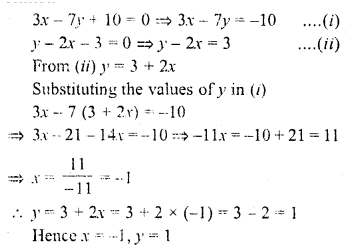Question 3 :
0.4x + 0.3y = 1.7
0.7x – 0.2y = 0.8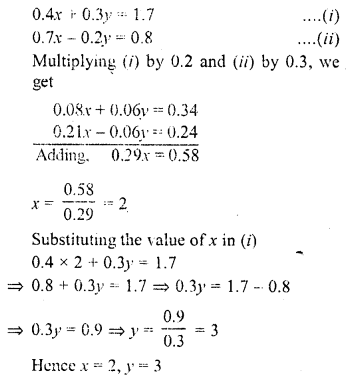Question 4 :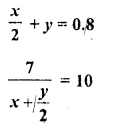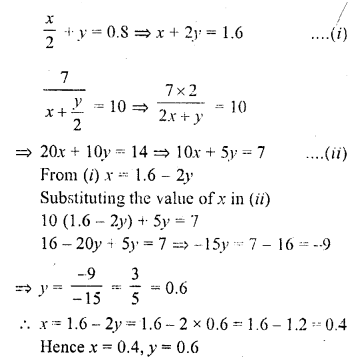Question 5 :
7(y + 3) – 2(x + 2) = 14
4(y – 2) + 3(x – 3) = 2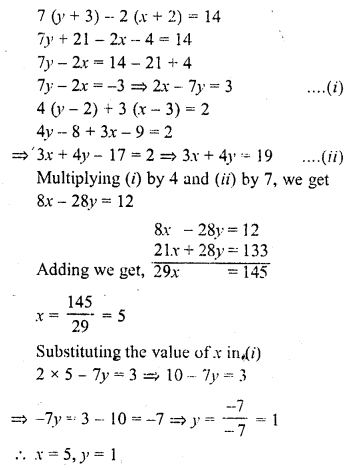Question 6 :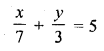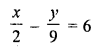Answer 6 :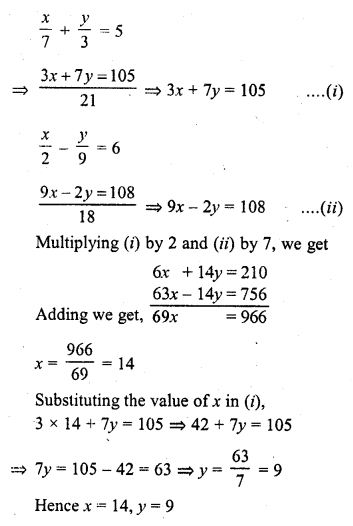Question 7 :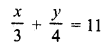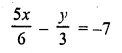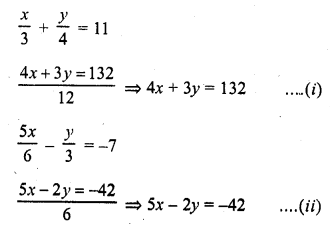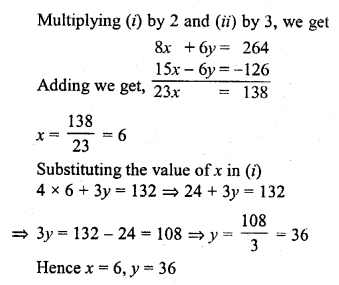Question 8 :
4/x + 3y = 8
6/x – 4y = -5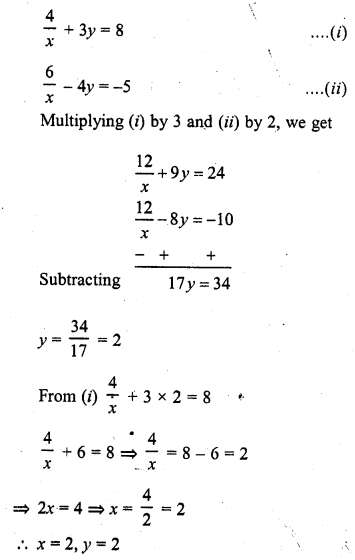Question 9 :
x + y/2 = 4
x/3 + 2y = 5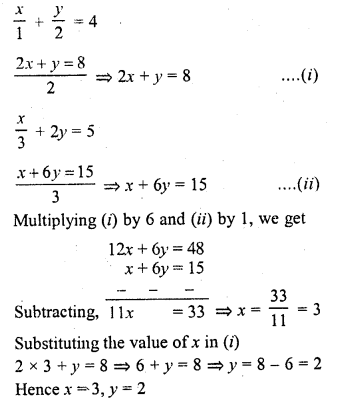Question 10 :
x + 2y = 3/2
2x + y = 3/2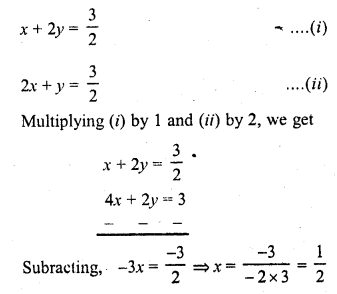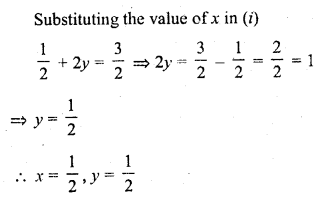Todays Deals### RD Chapter 3- Pair of Linear Equations in Two Variables Ex-3.3 Contributorskrishan

Name:
Email:

# Latest News# 9000 interview questions in different categories